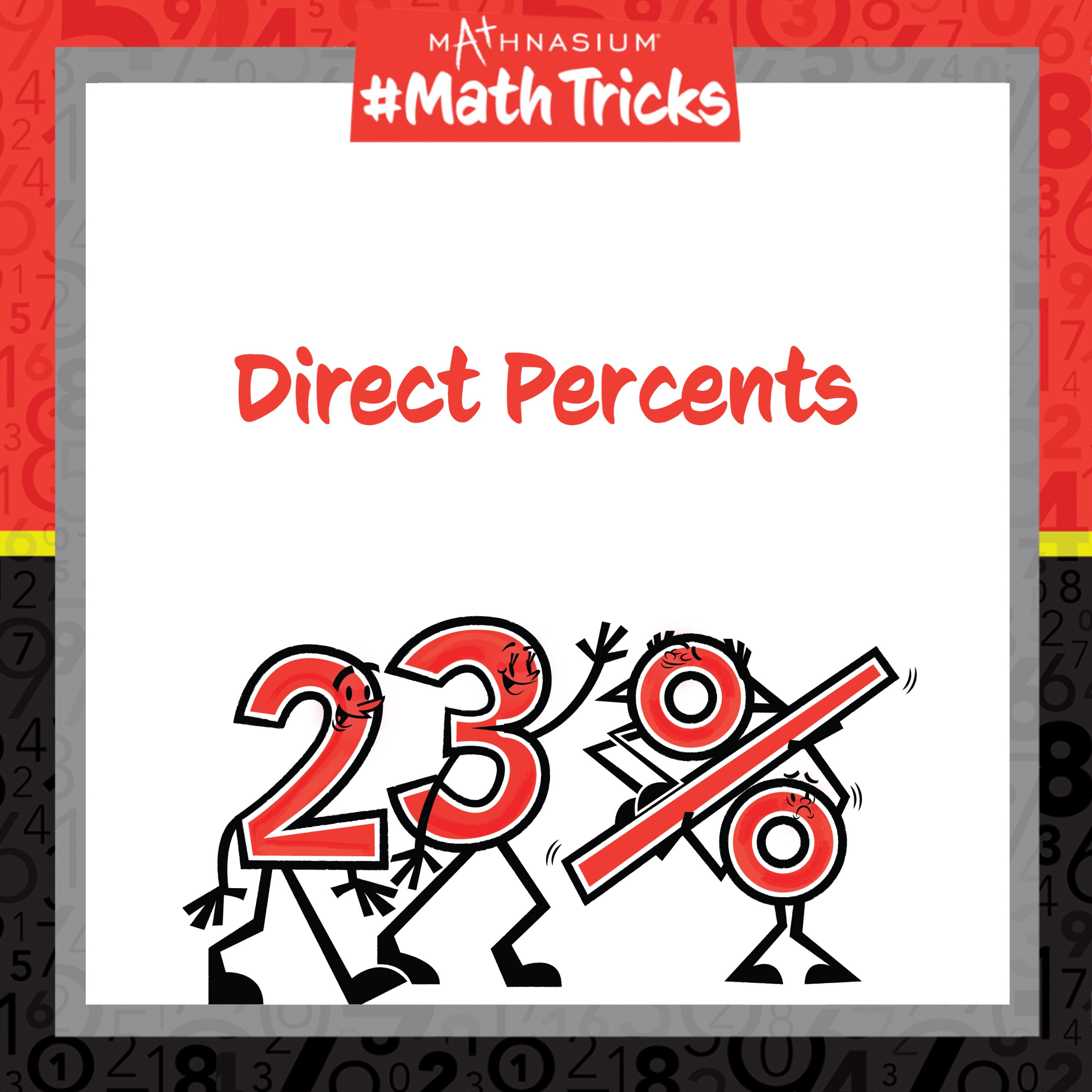877-601-6284
Get Started by Finding a Local Center

# Mathnasium #MathTricks: Direct Percents

May 31, 2023Welcome to Mathnasium’s Math Tricks series. Today we are finding the direct percentage amount of any number.

To calculate the “direct percent” of a number, we use the following steps:

1. Estimate the answer by rounding the number to the nearest multiple of 50.
2. Convert the percent to a decimal.
3. Multiply the number by the decimal.
4. Check for reasonableness.

Follow the example below to find the direct percent.

##### Example: It takes Larissa 363 total minutes to get from her house to her grandmother’s house. If 87% of this time is spent on an airplane, how many minutes does she spend on an airplane?

Step 1: Estimate the answer by rounding the number to the nearest multiple of 50.
Rounding the number 363 to the nearest multiple of 50, we get 350.
Since 87% means 87 for each 100, we estimate 87% of 350, which is equal to 304.5.

Step 2: Convert the percent to a decimal.
To convert 87% to a decimal we move the decimal point two places to the left, leaving us with 0.87.

Step 3: Multiply the number by the decimal.
Multiplying the number 363 by the decimal 0.87, we get an answer of 315.81.

Step 4: Check for reasonableness.
Our estimate was 304.5, so 315.81 is very reasonable.

Now, with this strategy, you are ready to use this Mathnasium Math Trick to find the direct percent of any number by converting the percent to a decimal and multiplying it by the number. Click here for more practice problems, then check your answers here.

If you missed this, or any of our other Math Tricks videos, check them out on our YouTube channel!

Do you have a math trick you want to see? Submit your request at: http://bit.ly/MathnasiumMathTricks.

## SEE HOW MATHNASIUM WORKS FOR YOUR SITUATION

### My Child is:## OUR METHOD WORKS

Mathnasium meets your child where they are and helps them with the customized program they need, for any level of mathematics.Early LearnersElementary SchoolMiddle SchoolHigh School